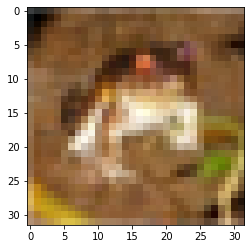Learn data science and machine learning by building real-world projects on Jovian

## Classifying images of everyday objects using a neural network

The ability to try many different neural network architectures to address a problem is what makes deep learning really powerful, especially compared to shallow learning techniques like linear regression, logistic regression etc.

In this assignment, you will:

1. Explore the CIFAR10 dataset: https://www.cs.toronto.edu/~kriz/cifar.html
2. Set up a training pipeline to train a neural network on a GPU
3. Experiment with different network architectures & hyperparameters

As you go through this notebook, you will find a ??? in certain places. Your job is to replace the ??? with appropriate code or values, to ensure that the notebook runs properly end-to-end. Try to experiment with different network structures and hypeparameters to get the lowest loss.

You might find these notebooks useful for reference, as you work through this notebook:

In :
``````# Uncomment and run the commands below if imports fail
# !conda install numpy pandas pytorch torchvision cpuonly -c pytorch -y
# !pip install matplotlib --upgrade --quiet``````
In :
``````import torch
import torchvision
import numpy as np
import matplotlib.pyplot as plt
import torch.nn as nn
import torch.nn.functional as F
from torchvision.datasets import CIFAR10
from torchvision.transforms import ToTensor
from torchvision.utils import make_grid
from torch.utils.data import random_split
%matplotlib inline
``````
In :
``````# Project name used for jovian.commit
project_name = '03-cifar10-feedforward'``````

### Exploring the CIFAR10 dataset

In :
``````dataset = CIFAR10(root='data/', download=True, transform=ToTensor())
test_dataset = CIFAR10(root='data/', train=False, transform=ToTensor())``````
```Downloading https://www.cs.toronto.edu/~kriz/cifar-10-python.tar.gz to data/cifar-10-python.tar.gz ```
``HBox(children=(FloatProgress(value=1.0, bar_style='info', max=1.0), HTML(value='')))``
```Extracting data/cifar-10-python.tar.gz to data/ ```

Q: How many images does the training dataset contain?

In :
``````dataset_size = len(dataset)
dataset_size
``````
Out:
``50000``

Q: How many images does the training dataset contain?

In :
``````test_dataset_size = len(test_dataset)
test_dataset_size
``````
Out:
``10000``

Q: How many output classes does the dataset contain? Can you list them?

Hint: Use `dataset.classes`

In :
``````classes = dataset.classes
classes
``````
Out:
``````['airplane',
'automobile',
'bird',
'cat',
'deer',
'dog',
'frog',
'horse',
'ship',
'truck']``````
In :
``````num_classes = len(classes)
num_classes
``````
Out:
``10``

Q: What is the shape of an image tensor from the dataset?

In :
``````img, label = dataset
img_shape = img.shape
img_shape
``````
Out:
``torch.Size([3, 32, 32])``

Note that this dataset consists of 3-channel color images (RGB). Let us look at a sample image from the dataset. `matplotlib` expects channels to be the last dimension of the image tensors (whereas in PyTorch they are the first dimension), so we'll the `.permute` tensor method to shift channels to the last dimension. Let's also print the label for the image.

In :
``````img, label = dataset
plt.imshow(img.permute((1, 2, 0)))
print('Label (numeric):', label)
print('Label (textual):', classes[label])``````
```Label (numeric): 6 Label (textual): frog ```(Optional) Q: Can you determine the number of images belonging to each class?

Hint: Loop through the dataset.

In :
``````class_dict = dict.fromkeys(classes, 0)
for img, label in dataset:
for class_label in classes:
if class_label==classes[label]:
class_dict[class_label] = class_dict[class_label] + 1

print("Number of Images Belonging to Each Class:\n")
for key, value in class_dict.items():
print(key,': ', value)``````
```Number of Images Belonging to Each Class: airplane : 5000 automobile : 5000 bird : 5000 cat : 5000 deer : 5000 dog : 5000 frog : 5000 horse : 5000 ship : 5000 truck : 5000 ```

Let's save our work to Jovian, before continuing.

In :
``!pip install jovian --upgrade --quiet``
In :
``import jovian``
In :
``jovian.commit(project=project_name, environment=None)``
```[jovian] Attempting to save notebook.. [jovian] Updating notebook "karthicksothivelr/03-cifar10-feedforward" on https://jovian.ml/ [jovian] Uploading notebook.. [jovian] Committed successfully! https://jovian.ml/karthicksothivelr/03-cifar10-feedforward ```
Out:
``'https://jovian.ml/karthicksothivelr/03-cifar10-feedforward'``

### Preparing the data for training

We'll use a validation set with 5000 images (10% of the dataset). To ensure we get the same validation set each time, we'll set PyTorch's random number generator to a seed value of 43.

In :
``````torch.manual_seed(43)
val_size = 5000
train_size = len(dataset) - val_size``````

Let's use the `random_split` method to create the training & validation sets

In :
``````train_ds, val_ds = random_split(dataset, [train_size, val_size])
len(train_ds), len(val_ds)``````
Out:
``(45000, 5000)``

We can now create data loaders to load the data in batches.

In :
``batch_size=128``
In :
``````train_loader = DataLoader(train_ds, batch_size, shuffle=True, num_workers=4, pin_memory=True)
Let's visualize a batch of data using the `make_grid` helper function from Torchvision.
``````for images, _ in train_loader:
```images.shape: torch.Size([128, 3, 32, 32]) ```